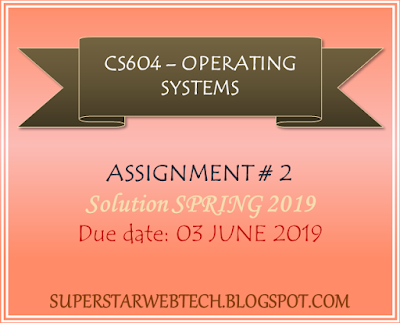## Monday, 3 June 2019

### CS604-Operating Systems Assignment no. 2 Solution SPRING 2019 Due date: 03 June, 2019 | SUPERSTARWEBTECH

Subject: CS604-Operating Systems
Semester: SPRING 2019
Assignment No. 2
Due date: 03 JUNE 2019CS604 ASSIGNMENT 2 SOLUTION SPRING 2019

CS604-Operrating Systems Assignment 2 #Question

Question 1:
Assume you have to apply First Come First Serve (FCFS) scheduling algorithms on the set of different processes given in the table below. The CPU burst time is also given for each process. Consider that all the processes arrive in the ready queue within time 0 seconds. You are required to show the Gantt chart to illustrate the execution sequence of these processes and calculate the total waiting time and average waiting time for the given processes by using FCFS algorithm.
Question 2:

Consider a scenario where you have to apply the Round Robin scheduling algorithm on the below-given set of processes with each having a quantum size=10 milliseconds. The CPU burst time and arrival time for each process are also provided in the given table. You are required to show the Gantt chart to illustrate the execution sequence of these processes. Moreover, calculate the average turnaround time and average waiting time for given processes by using a round robin algorithm.

To understand the Round-Robin scheduling algorithm watch this tutorial: